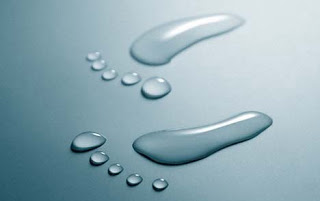# Calculating Total Body WaterThe easy way to calculate total body water is simply to multiply 0.6 times your weight in kilograms, since roughly 2/3 of your body weight is water.
There are fancier (and more accurate) equation-based calculations used to determine total body water as well.  These include the Watson formula, the Hume formula, and the Mellits-Cheek formula (specifically designed for use in children).  A handy link to a calculator using these formulas can be found here.
How were these formulas arrived at?  For the calculation of the Watson formula, for instance, dilutional methods using deuterated water (a.k.a. “heavy water”) were carried out in order to obtain a “gold standard” of total body water for a large population of normal individuals; statistics were then applied to generate an equation which accurately predicts TBW based on variables such as weight, height, age, and gender.  To carry out such a study, a known dose of heavy water (D2O) is ingested by healthy volunteers and allowed to equilibrate with total body water.  Then, a mass spectrometry instrument is used to measure the deuterium:hydrogen ratio in exhaled body water vapor; the total body water can then be calculated by determining the increase in breath deuterium content in relation to the volume of heavy water taken in.  An alternative technique to determining TBW is using bioelectrical impedence analysis.

### 1 comment

1.My water capacity